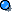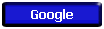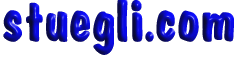#Energy Crossword

Complete the crossword puzzle.Across 4. force times distance  (SI - Joules = N-m) 1 J = 1 N x 1 m 5. work per unit time (work divided by time) 7. will raise 1 gram 1 degree Celsius 9. energy of motion (mass times the square of the velocity) 11. electromagnetic waves that directly transport energy through space 12. the ability to do work Down 1. the sum of kinetic and potential energies in an object 2. unit of energy (force times distance) 3. stored energy, energy of position 6. the energy that flows from one object to another due to differences in temperature 7. the transfer of heat energy in a gas or liquid by movement of currents 8. the transfer and distribution of heat energy from atom to atom within a substance 10. SI unit of power  (work/time)Copyright © 2005 -  S. B. EglI